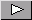Centrale des maths - centraledesmaths.uregina.caCentre de ressources« centre de ressources »VoletSecondaire -La trigonométrienouvelle recherche

Nous avons trouvé 22 articles correspondant à votre recherche.Page1/2Géographie rencontre mathématiques à mi-chemin entre les latitudes. AUTEUR(S): Oleksandr G. AkulovIn this note Gregory describes a problem involving Dasher and Dancer moving around a Northern Light Circle. AUTEUR(S): Gregory V. AkulovAn example of a problem in algebra or trigonometry that is motivated by an exercise with a graphing calculator. The graph leads to an exercise with a trigonometric identity. AUTEUR(S): Rick SeamanIn this note the authors give an expression for locating the midpoint of a circular arc and a calculator for determining the midpoint. AUTEUR(S): Gregory V. Akulov and Oleksandr (Alex) G. AkulovIn this note the authors give an proof of the expression for locating the midpoint of a circular arc that was given in his note with Gregory V. Akulov. AUTEUR(S): Oleksandr (Alex) G. AkulovGregory and Oleksandr have built on the arc midpoint resource and the proof of the arc midpoint formula by constructing an algorithm for finding the coordinates of the midpoint. It is hoped that teachers of high school Mathematics and Computer Science will use these resources to enrich the teaching and learning in both subject areas. AUTEUR(S): Oleksandr G. Akulov and Gregory V. AkulovGregory and Oleksandr use their Arc Midpoint Computation approach to solve a problem concerning gravitational potential energy and then challenge the reader to solve the same problem using an alternative approach. AUTEUR(S): Gregory V. Akulov and Oleksandr G. AkulovGregory and Oleksandr extend their arc midpoint computation to determine the midpoint of a section of a sine curve. AUTEUR(S): Gregory V. Akulov and Oleksandr G. AkulovGregory finds another application of his arc midpoint computation, this time to the kinetic energy of an object moving along a semicircle. AUTEUR(S): Gregory V. AkulovOleksandr and Gregory extend interpretations of non-piecewise identities for sin^(-1)x+ sin^(-1)y and cos^(-1)x+ cos^(-1)y using several Euclidean geometry statements and illustrations. AUTEUR(S): Oleksandr G. Akulov and Gregory V. AkulovThis note is a response to a teacher's request for an explaination of i squared and the square root of i that would be appropriate for secondary level students. AUTEUR(S): Harley WestonIn this note Gregory uses a trig identity to develop an expression for the slopes of the angle bisectors of two lines in terms of the slopes of the lines that form the angle. AUTEUR(S): Gregory V. AkulovA trigonometric identity is used to develop a formula for the slope of a rhombus diagonal. This expression is then used to find the velocity of a whale. AUTEUR(S): Gregory V. Akulov and Oleksii V. AkulovGregory and Oleksandr find more compact and efficient ways to express some identities involving arcsine and arccosine that appear in the Handbook of Mathematics. The expression Gregory and Oleksandr found was used to derive their arc midpoint computation. AUTEUR(S): Gregory V. Akulov and Oleksandr G. AkulovIn this note Gregory uses his Arc Midpoint Computation formula to devise a problem regarding riding a bicycle around the University of Victoria campus. AUTEUR(S): Gregory V AkulovPage1/2Centrale des maths reçoit une aide financière de l’Université de Regina et de The Pacific Institute for the Mathematical Sciences.Qui sommes-nous :: carte du site :: our english site